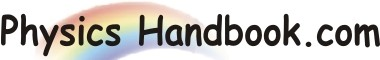HOME TOPICS DEFINITIONS TABLES LAWS INVENTIONS EXPERIMENTS QUIZ VIDEOS
 Newton's Third Law To every action there is always opposed an equal reaction; or, the mutual actions of two bodies upon each other are always equal, and directed to contrary parts. Let two bodies A and B that are interacting. We define FA as the force applied to body A by body B and FB as the force applied to body B by body A. These forces will be equal in magnitude and opposite in direction. In mathematical terms, it is expressed as: FB  =  - FA or   FA + FB  =  0 Newton's Third Law of Motion states that any time a force acts from one object to another, there is equal force acting back on the original object. If you pull on a rope, therefore, the rope is pulling back on you as well. For example, if the road exerts a forward frictional force on an accelerating car's tires, then it is also a frictional force that Newton's third law predicts for the tires pushing backward on the road.